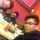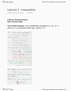# MATA33H3 Study Guide - Quiz Guide: Level Set, Partial Derivative, Farad

170 views17 pagespinkox631 and 3 others unlocked3
Verified Note
3 documents

## Document Summary

Topics included: multi-variable derivatives, level curves, partial derivatives and its applications, second order partial derivatives, high order partial derivatives, chain rule. Some common examples of several variables functions: recall the objective function in linear programming, where x,y are variables and z = f(x,y) = ax + by, where a,b are constants, and z is a real output. As the equation of the plane in general form is ax + by + cz = d you can then solve for it in terms of z giving f (x,y) = ax + by + d. In order to graph a plane you can generally find the intersection points with the three axes and then graph the triangle that connects those three points. This triangle" will be a portion of the plane and it will be a sizable visual graph looks like. Graph the plane given by f (x,y) = 12-3x-4y.